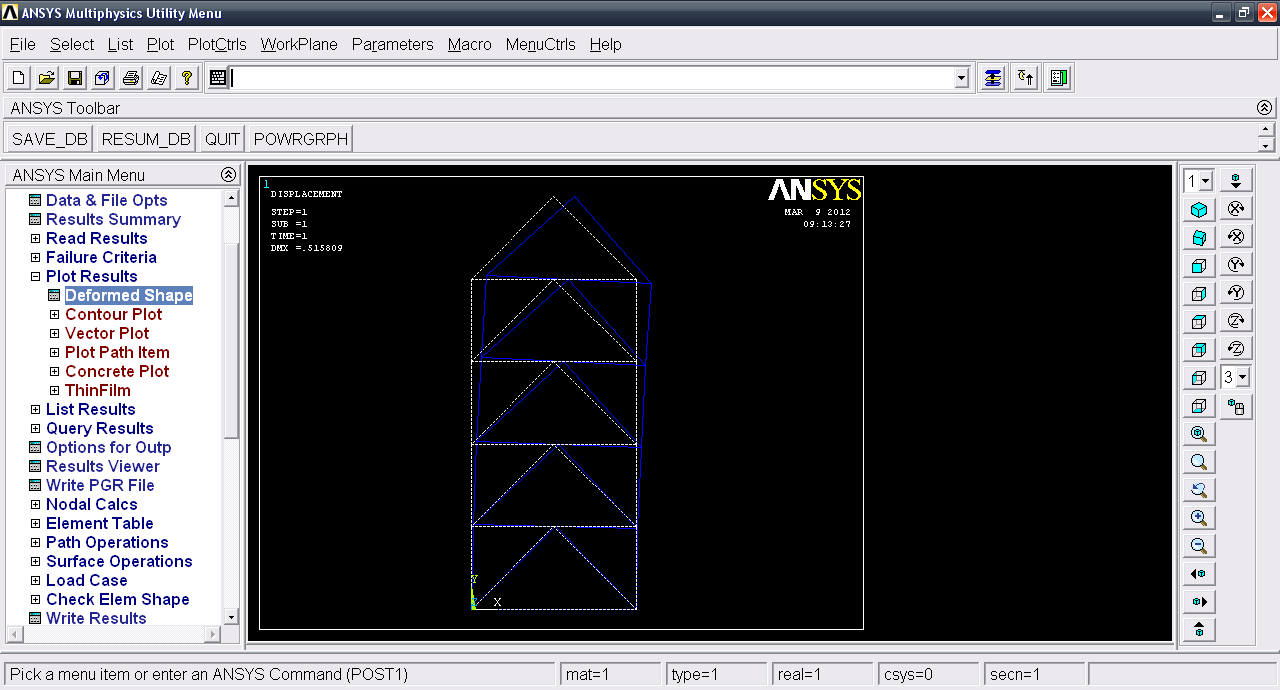# Tutorial - Solving 2D Truss problem using Mechanical APDL (ANSYS)?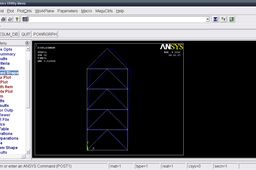In general, a finite element solution may be broken into the following three stages.

1. Preprocessing: defining the problem;
- Define keypoints/lines/areas/volumes
- Define element type and material/geometric properties
- Mesh lines/areas/volumes as required

2. Solution: assigning loads, constraints and solving;

3. Postprocessing:
- Lists of nodal displacements
- Element forces and moments
- Deflection plots
- Stress contour diagrams

We will go through these and solve the problem. I will suggest you to go through this tutorial before solving this problem. http://grabcad.com/questions/tutorial-2d-truss-analysis-in-mechanical-apdl-ansys-part-1

1. ### Step 1:

Start ANSYS Mehchanical APDL.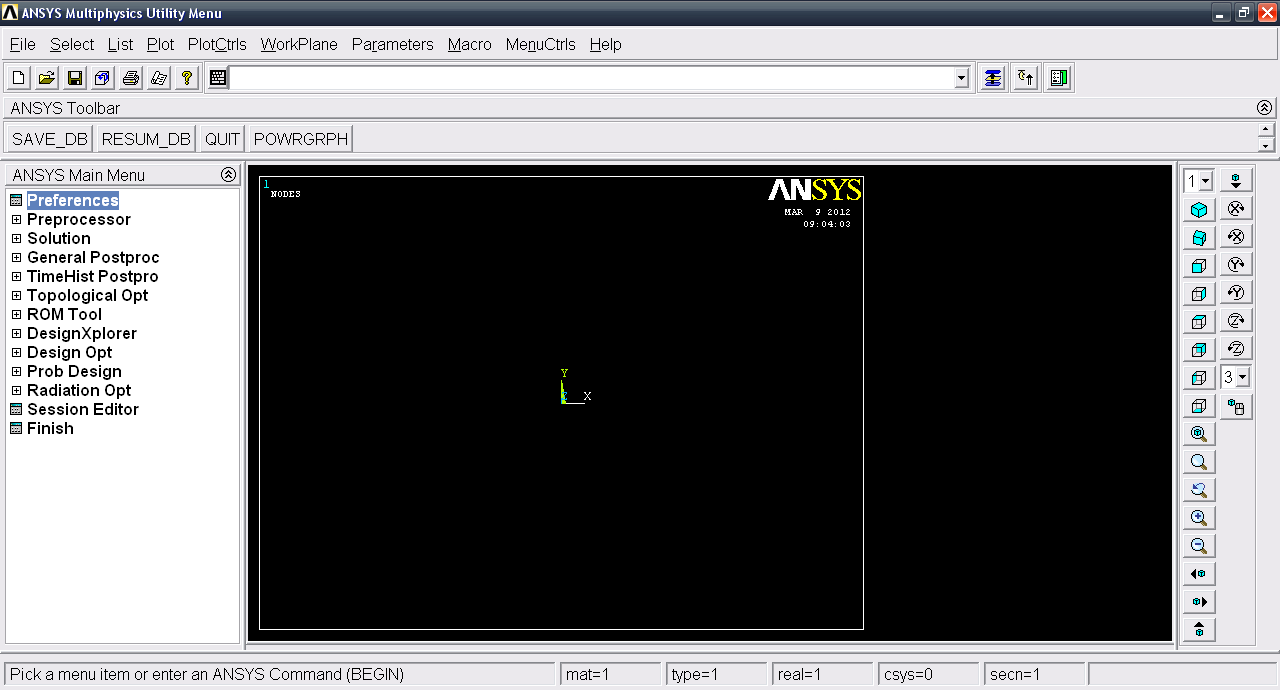2. ### Step 2:

Click Preferences and select Structural & click OK.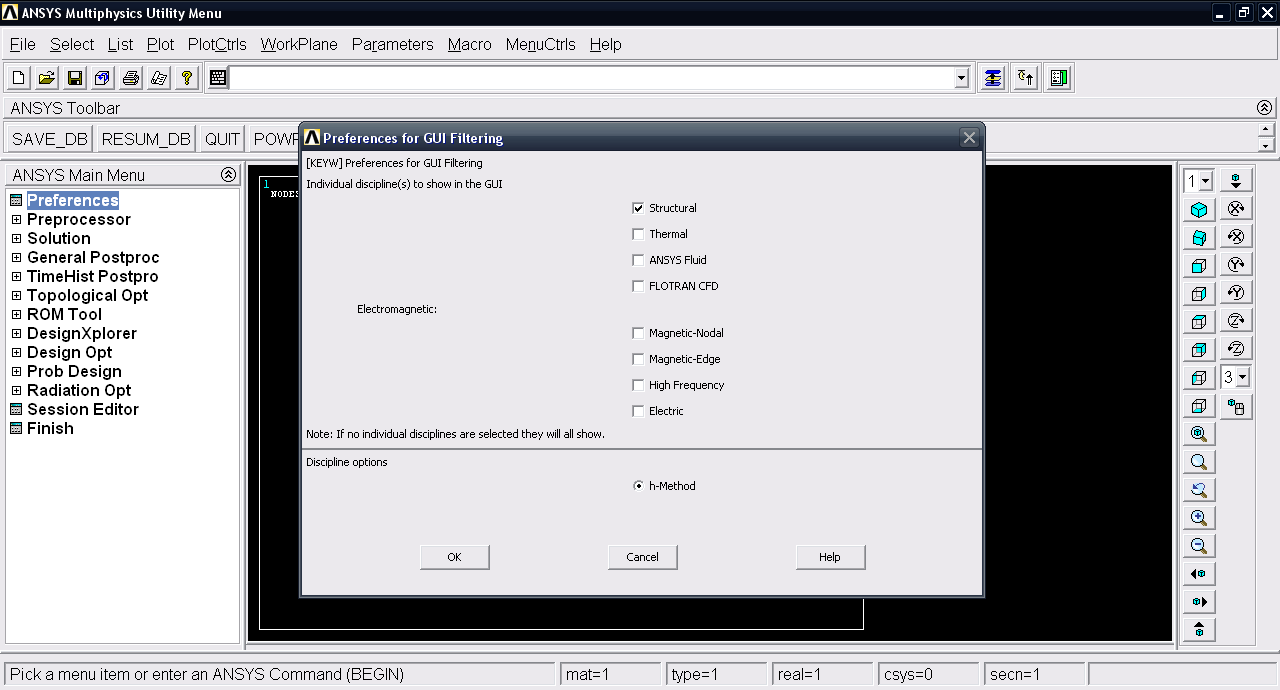3. ### Step 3:

Now we have the first step i.e. Preprocessing. Goto Modeling >> Create >> Keypoints >> In active CS. We will now create Keypoints for the problem.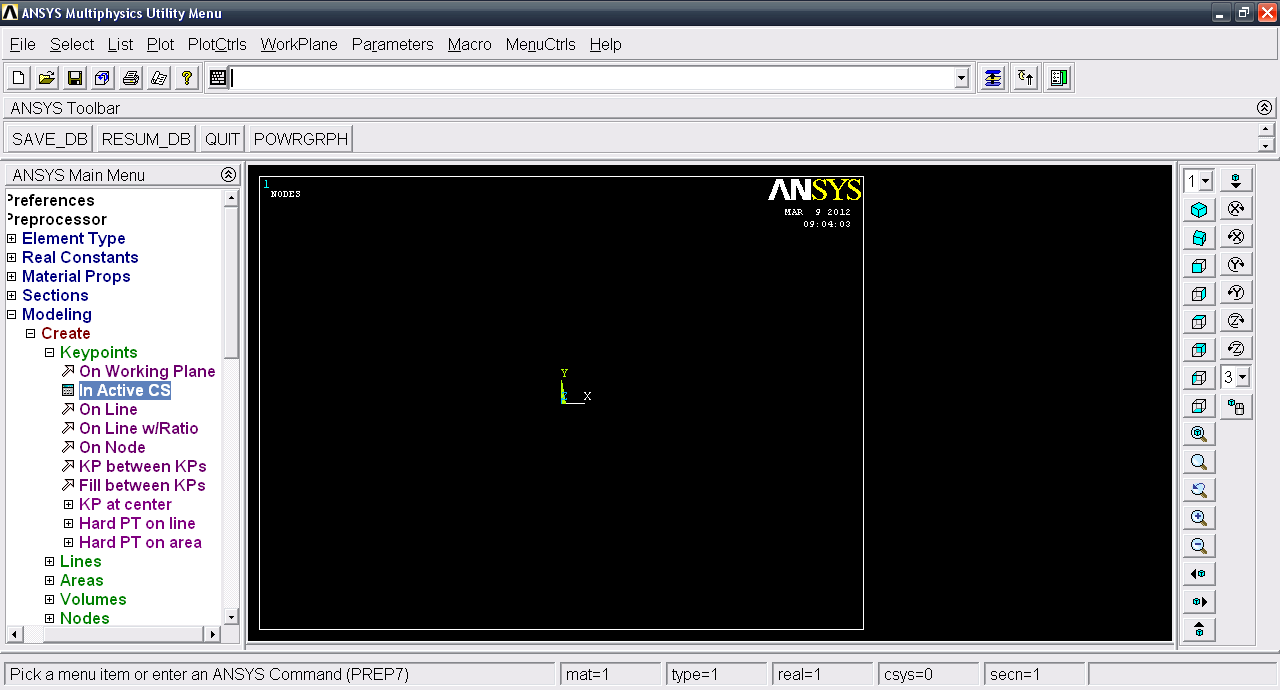4. ### Step 4:

Input keypoints
x y z
1. 0 0 0
2. 3000 3000 0
3. 6000 0 0
4. 0 3000 0
5. 6000 3000 0
6. 3000 6000 0
7. 0 6000 0
8. 6000 6000 0
9. 0 9000 0
10. 3000 9000 0
11. 6000 9000 0
12. 0 12000 0
13. 3000 12000 0
14. 6000 12000 0
15. 3000 15000 0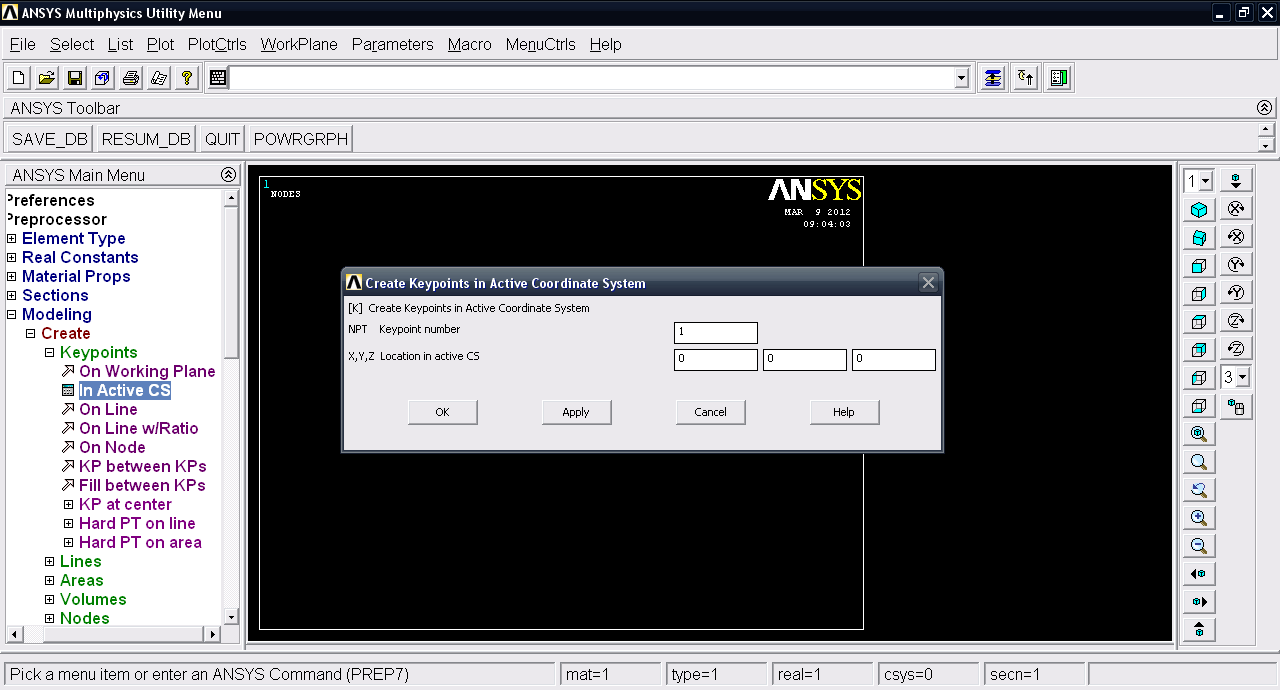5. ### Step 5:

We have the keypoints plotted.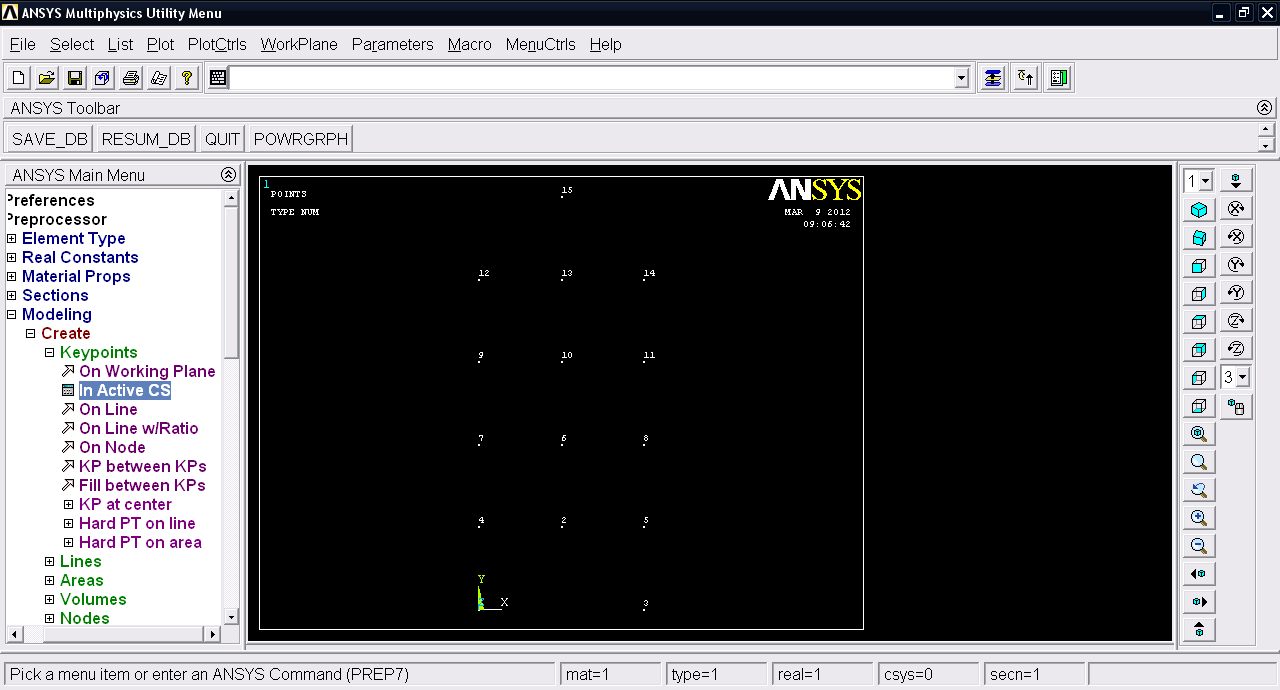6. ### Step 6:

We have to create the lines. Goto Modeling >> Create >> Lines >> Lines >> in active Coord.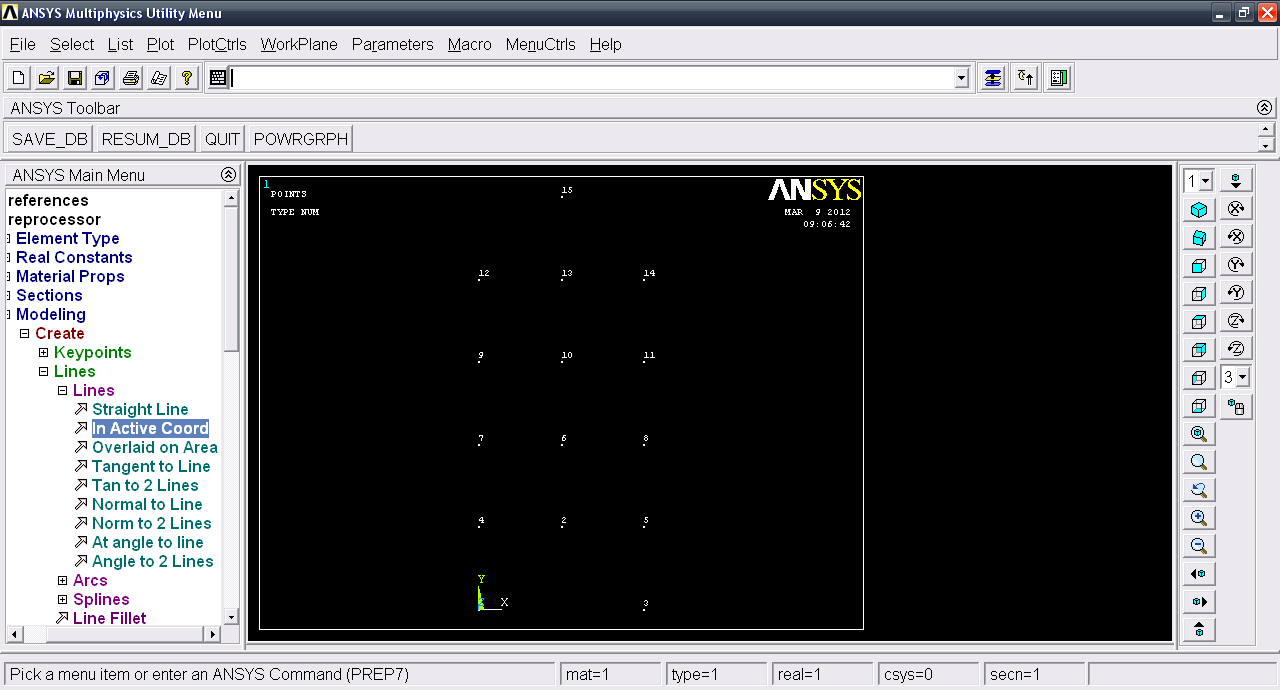7. ### Step 7:

Create lines. Click OK.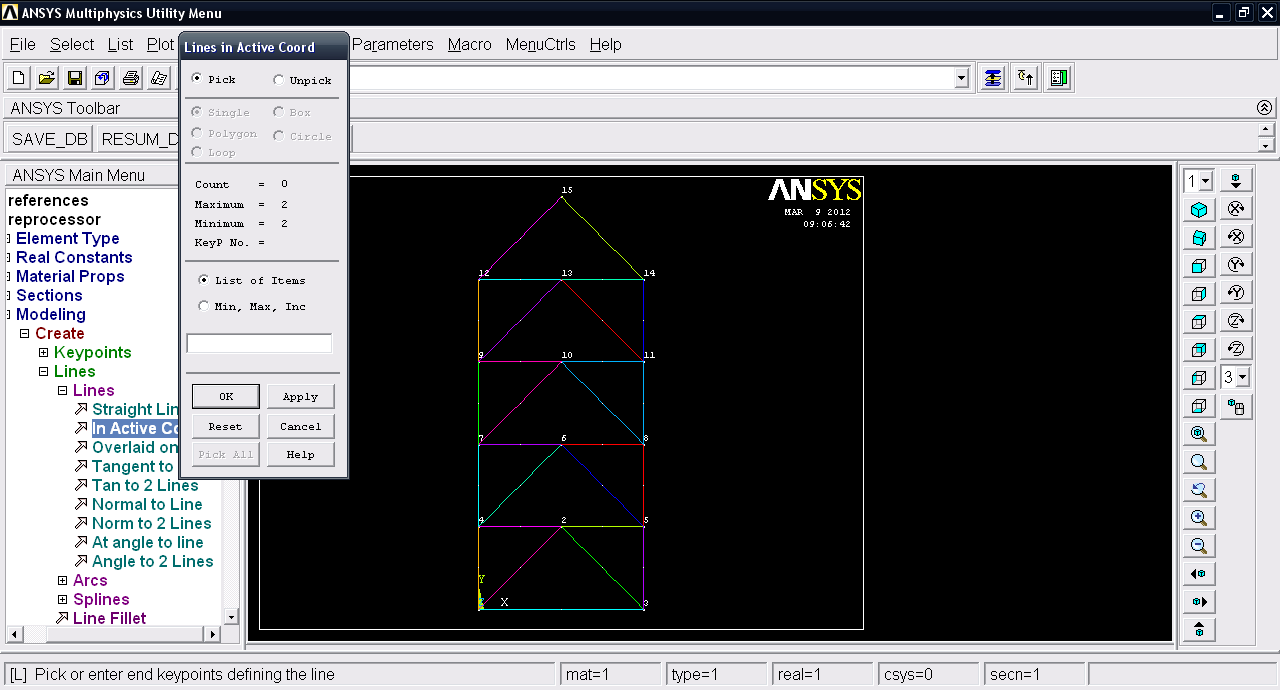8. ### Step 8:

Now we have to Define the mesh size. Goto >> Meshing >> Size Cntrls >> Manual Size >> Lines >> All Lines.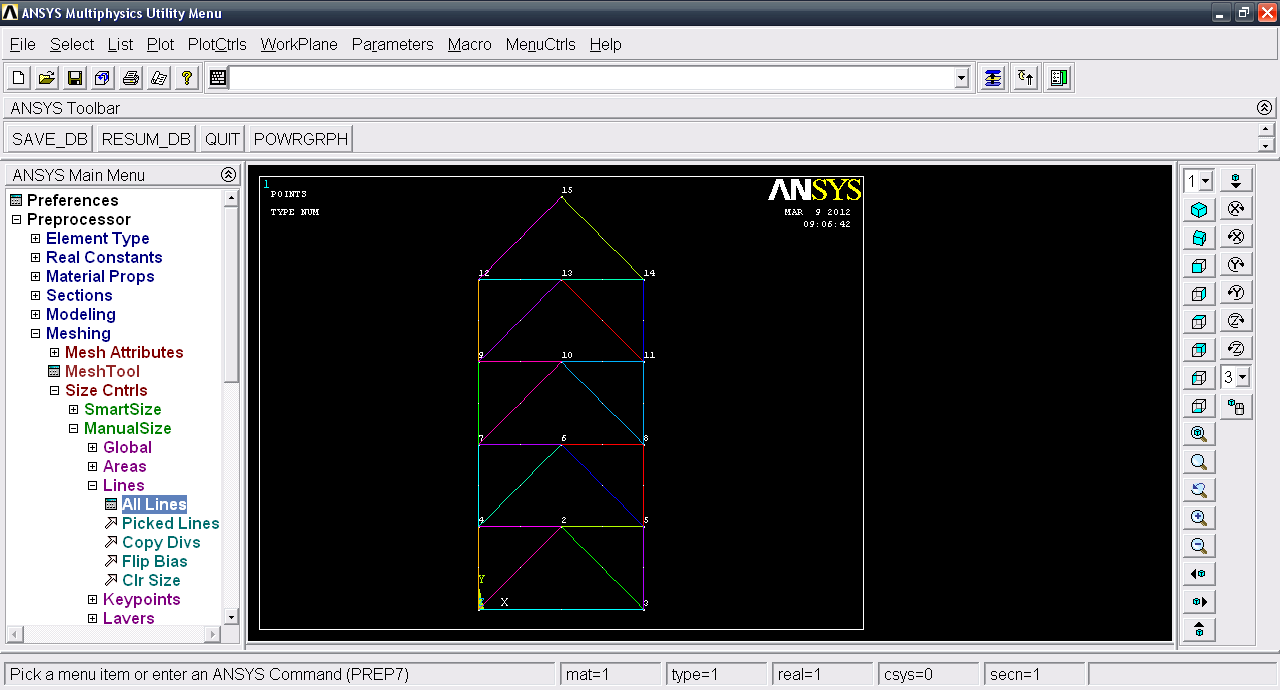9. ### Step 9:

Input number of element divisions = 1 and click OK.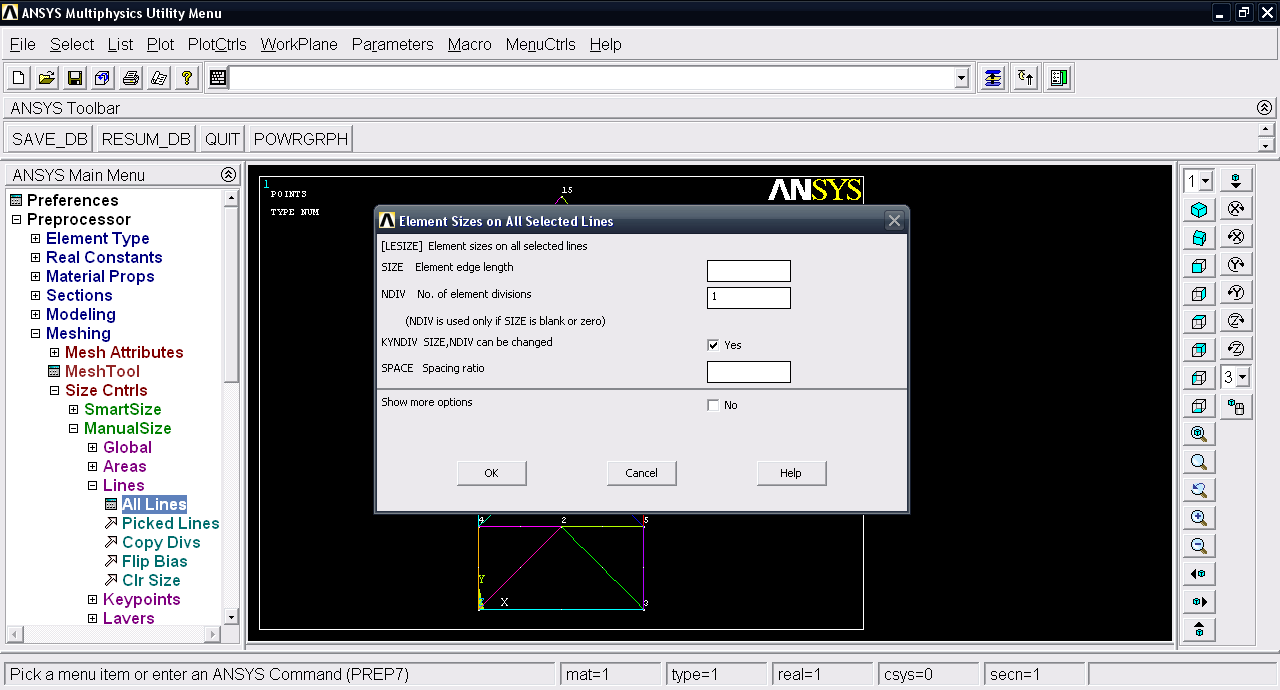10. ### Step 10:

Now we have to define the element type. Goto Element type >> Add/Edit/Delete.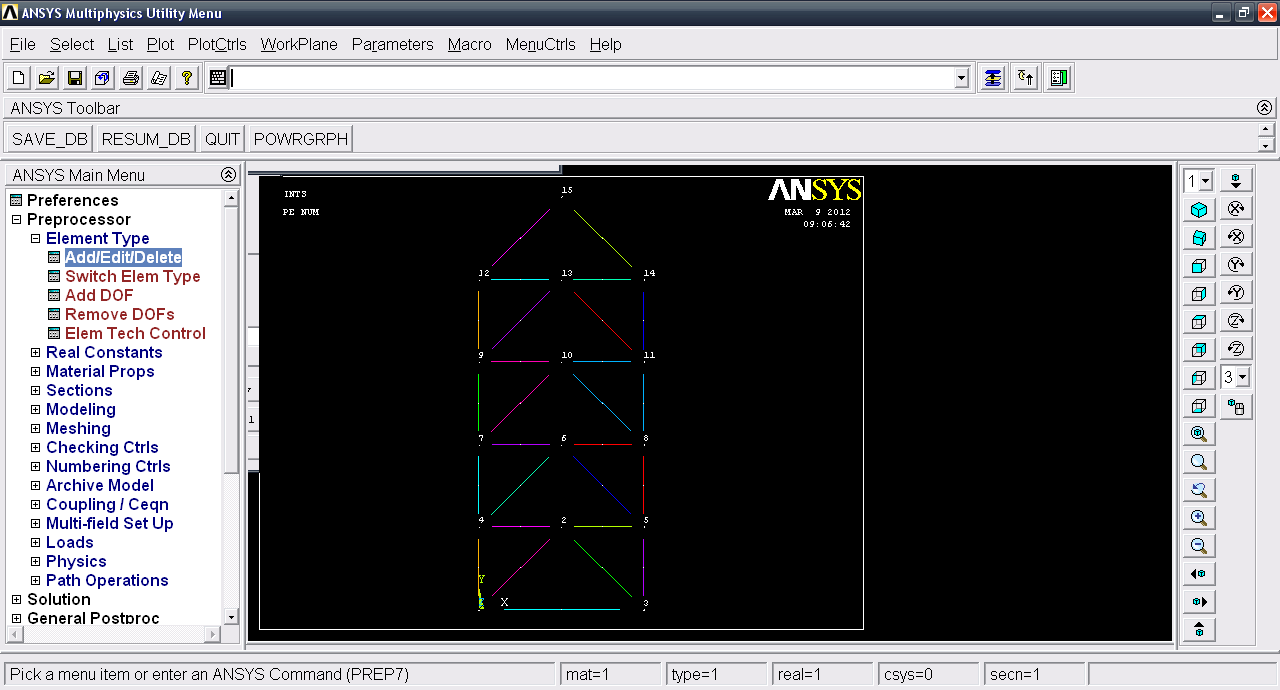11. ### Step 11:

Click Add and then select Link >> 3D finit stn 180. This is link 180. Click OK and then close.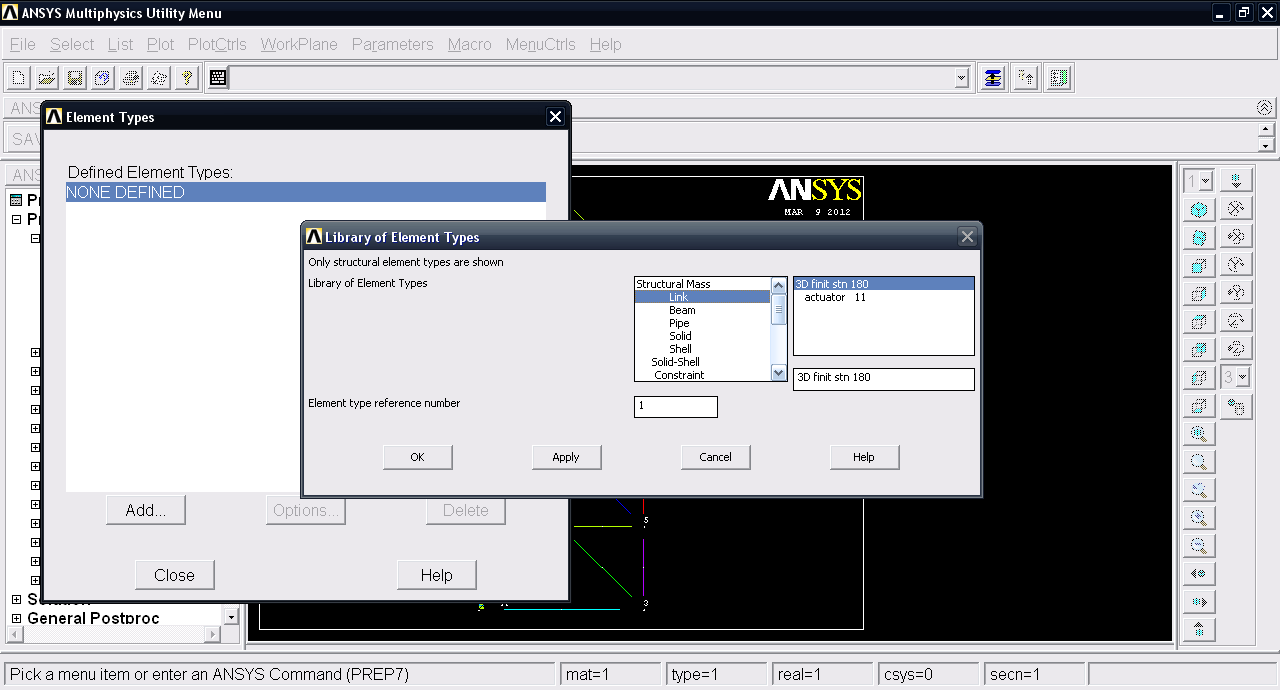12. ### Step 12:

Now we have to define the cross section of the truss. Goto Real Constants >> Add/Edit/Delete.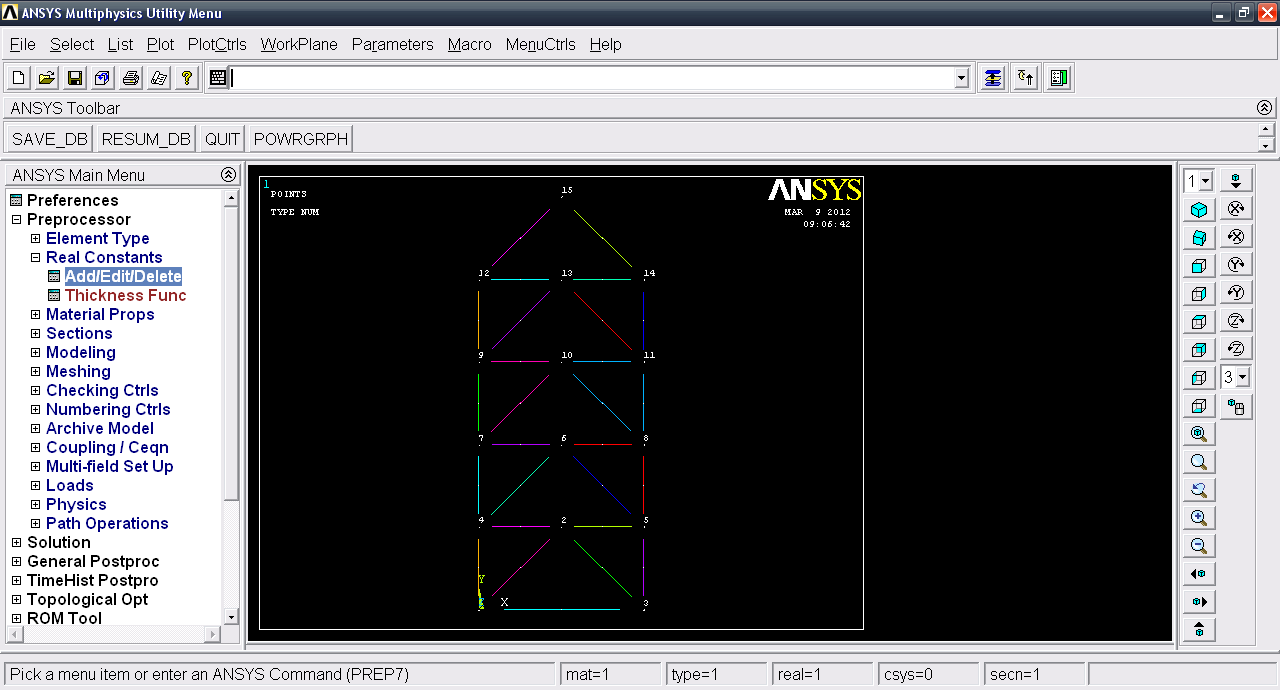13. ### Step 13:

Click Add and then define Cross-sectional area = 3500.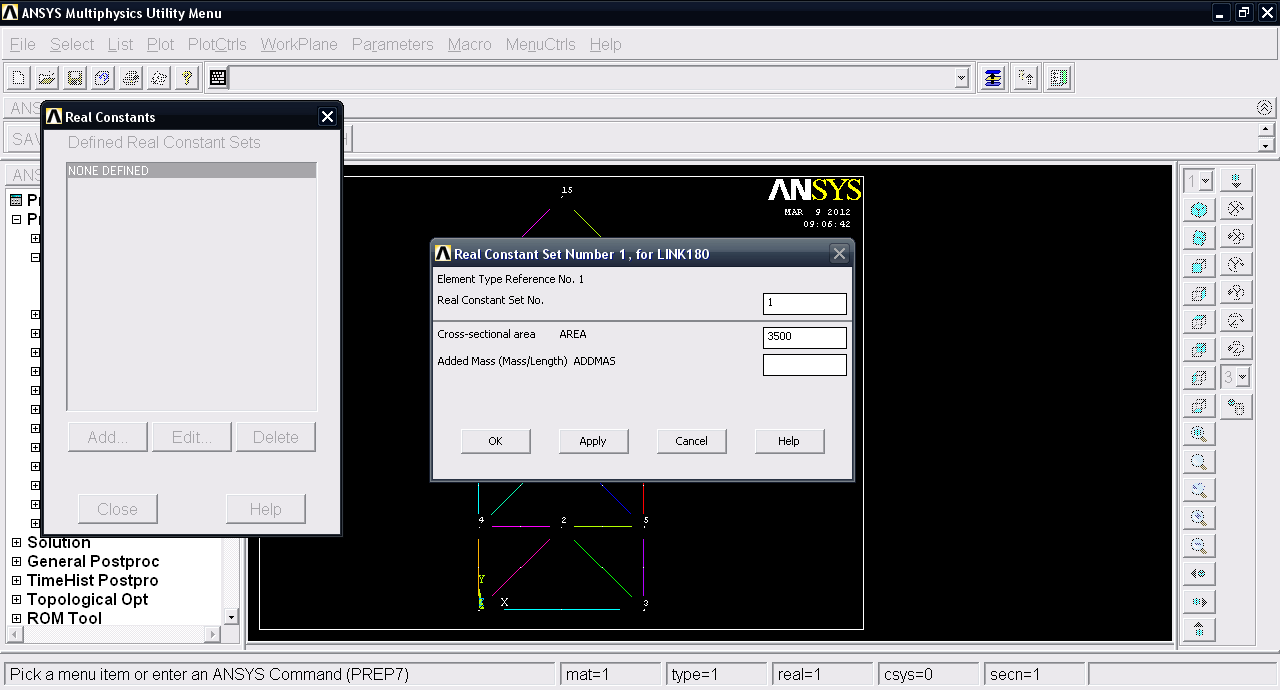14. ### Step 14:

Now we will create the mesh of this truss. Goto Meshing >> Mesh >> Lines.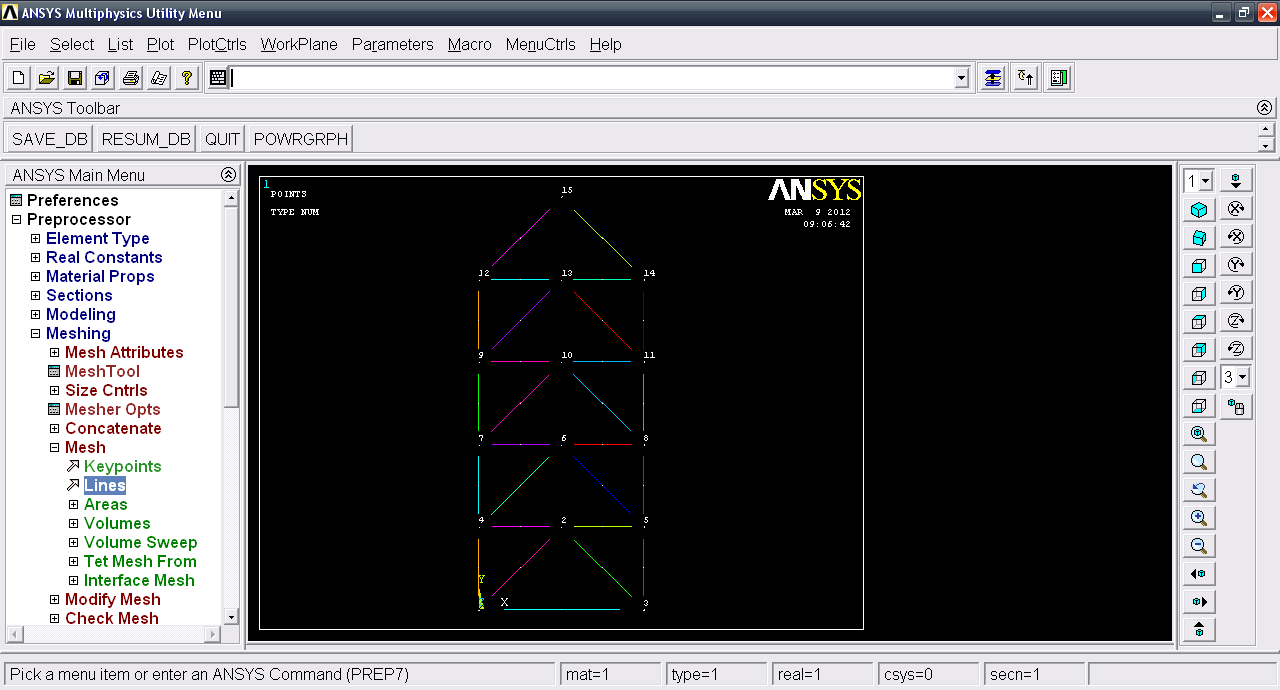15. ### Step 15:

Click Pick all.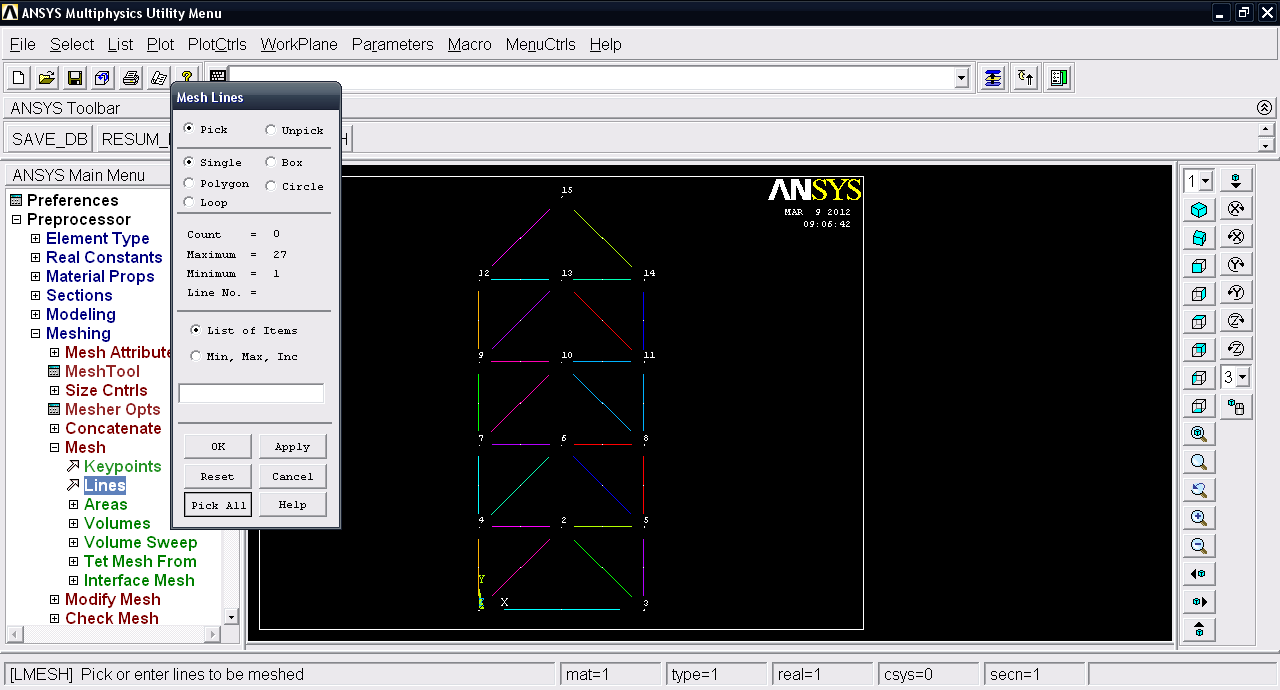16. ### Step 16:

Now we have to define the material properties. Goto Material Props >> Material models.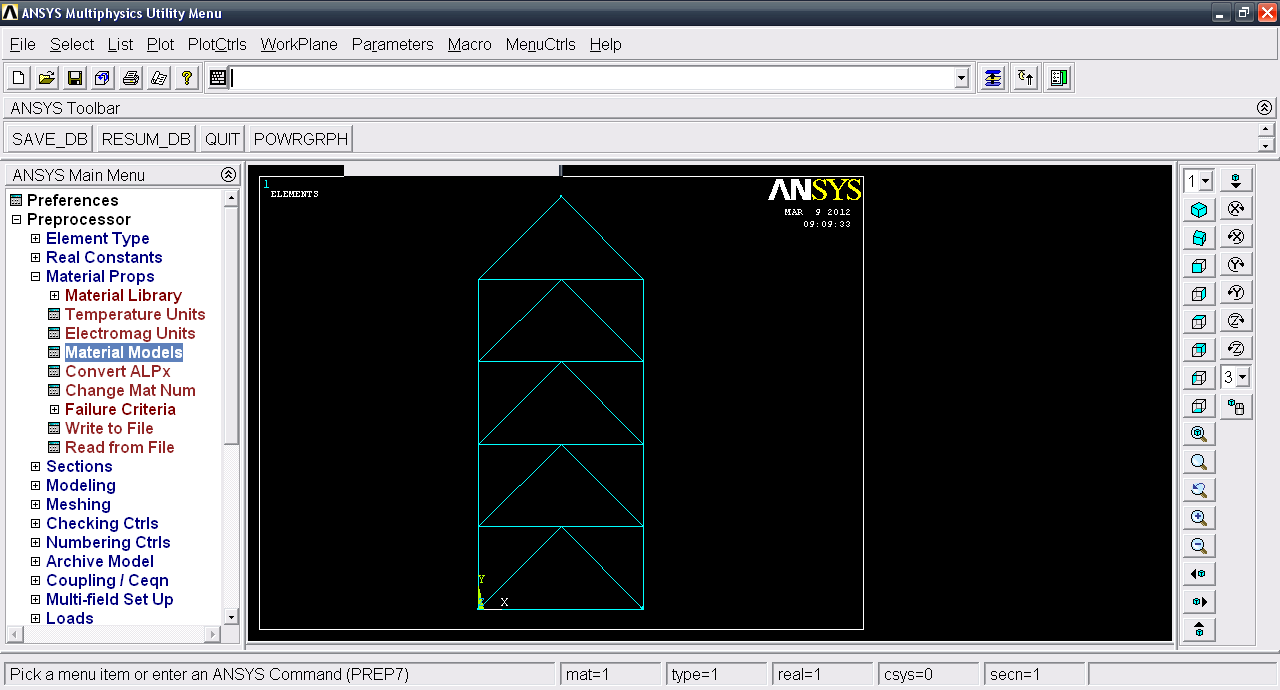17. ### Step 17:

Goto Structural >> Linear >> Elastic >> Isotropic.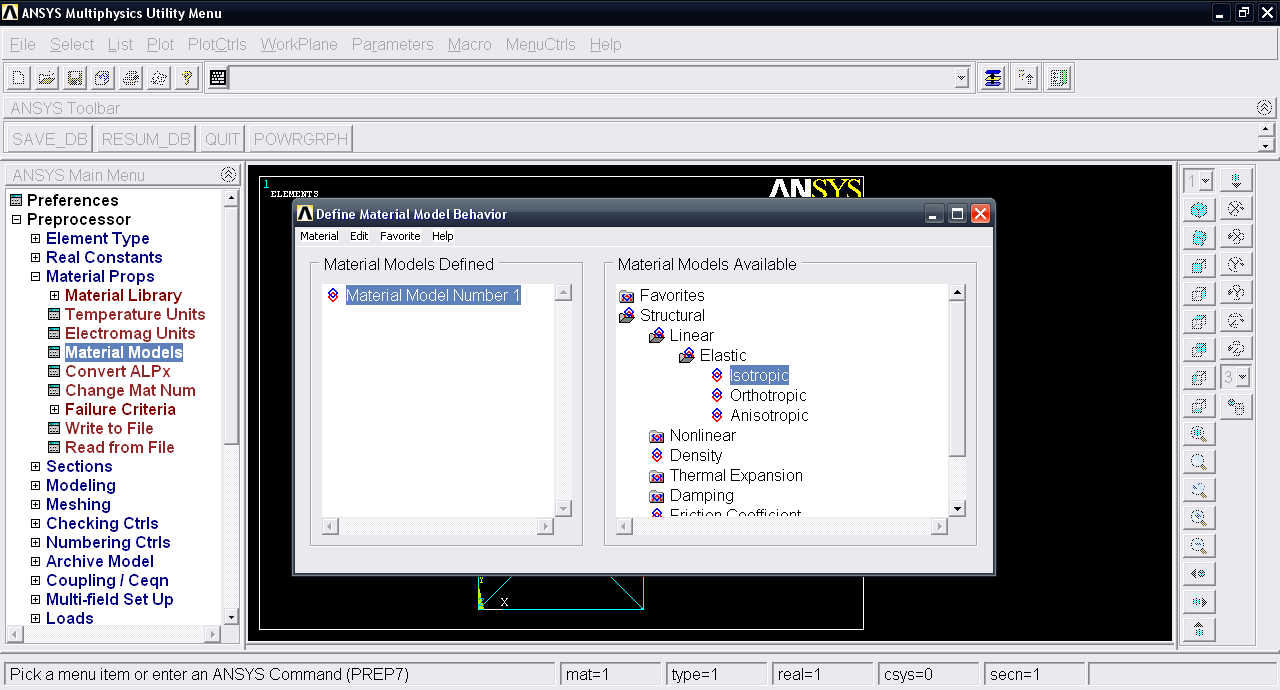18. ### Step 18:

Input EX=200000, PRXY=0. Click OK and then close.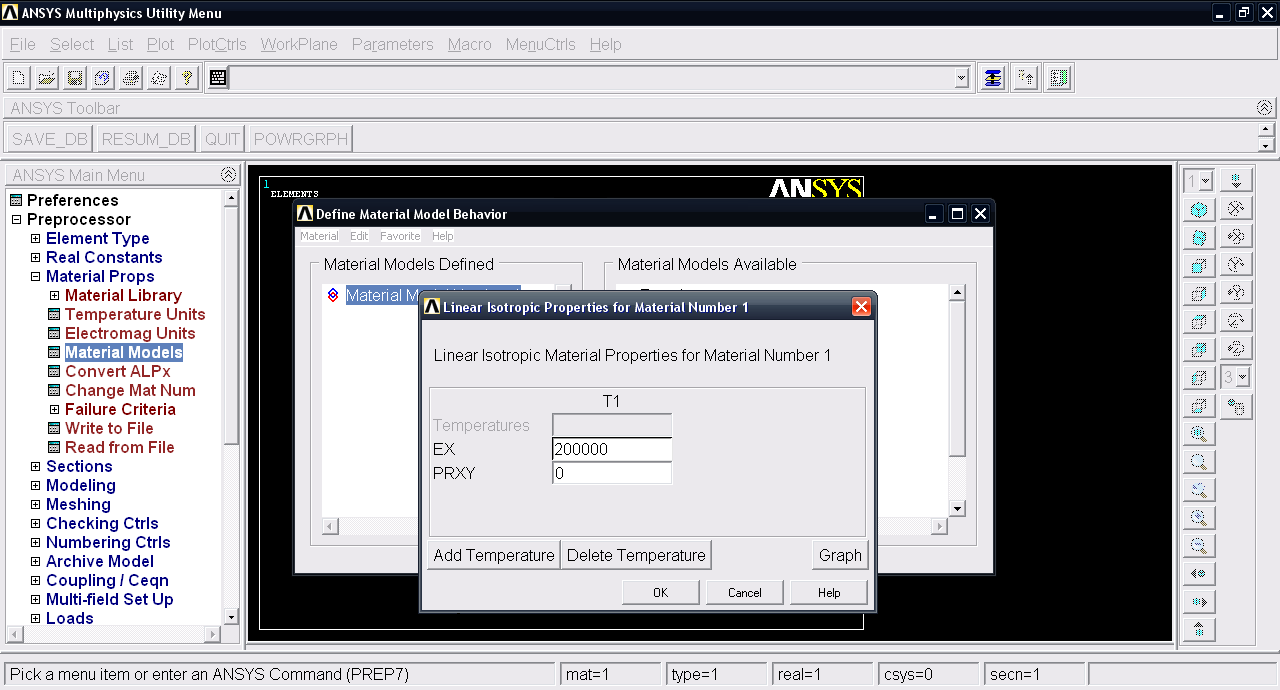19. ### Step 19:

Now the second step i.e. Solution. Goto Solution >> Analaysis Type >> New Analysis.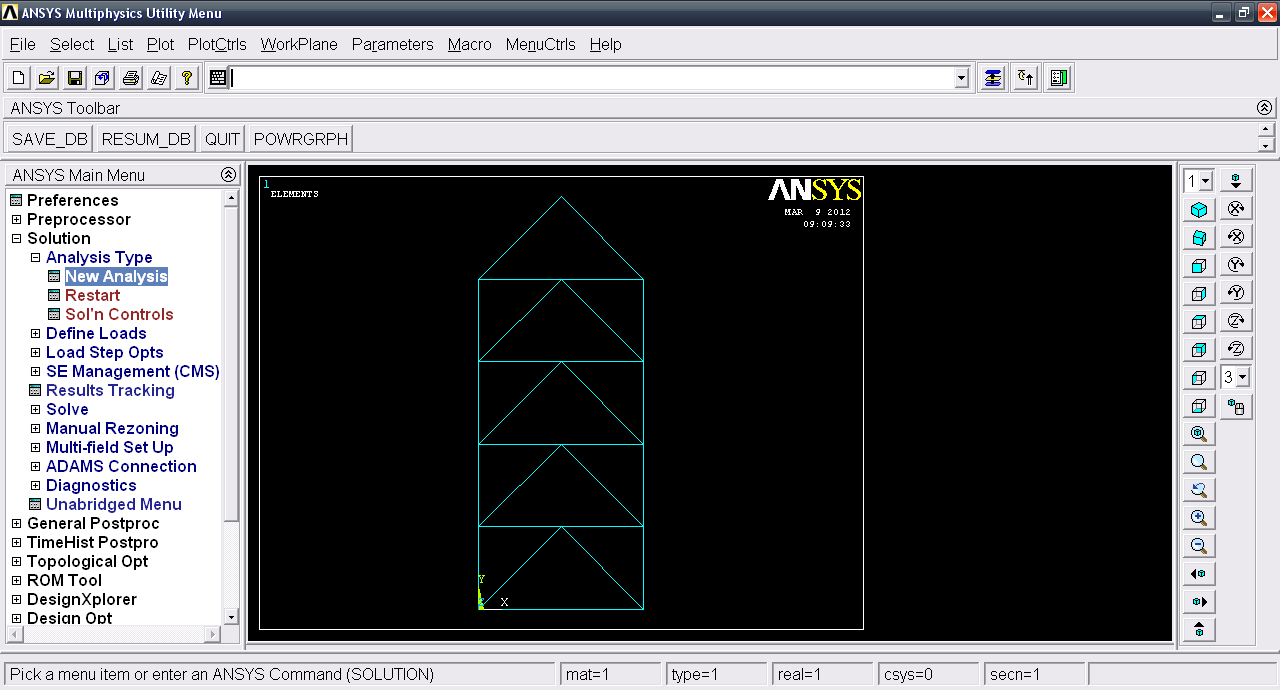20. ### Step 20:

Select Static and then click OK.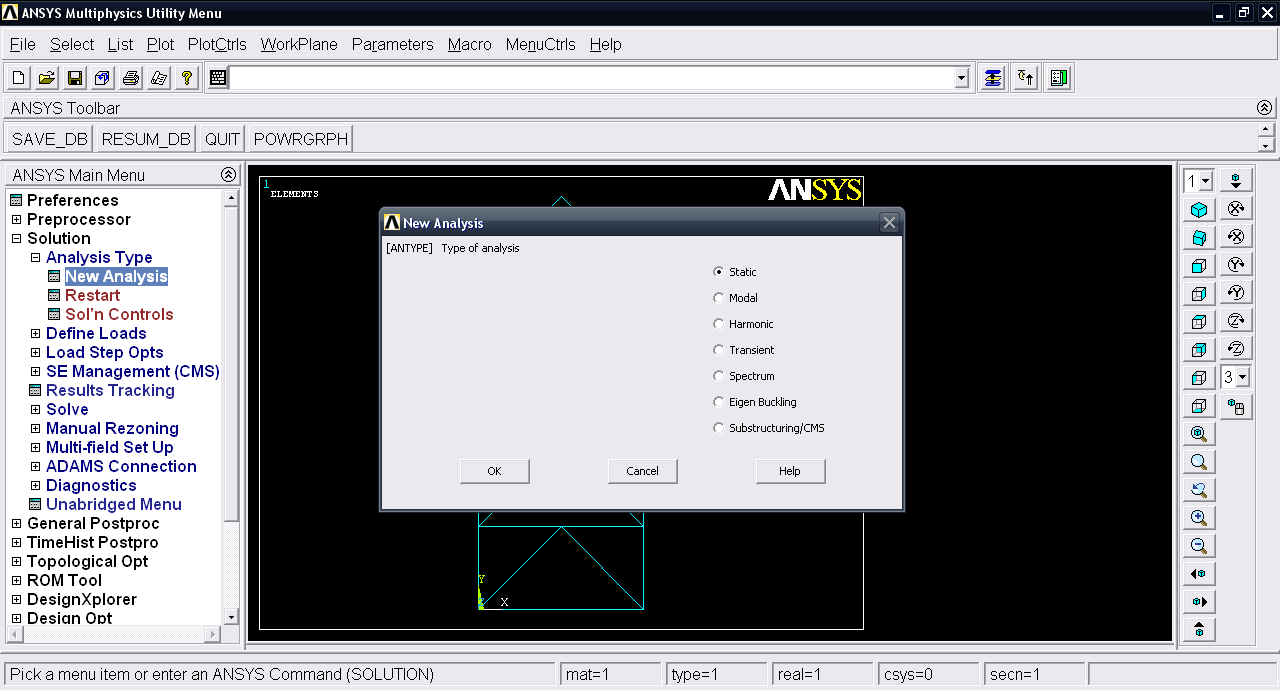21. ### Step 21:

Now we will define the fixed supports. Goto Define loads >> Apply >> Structural >> Displacement >> On Keypoints.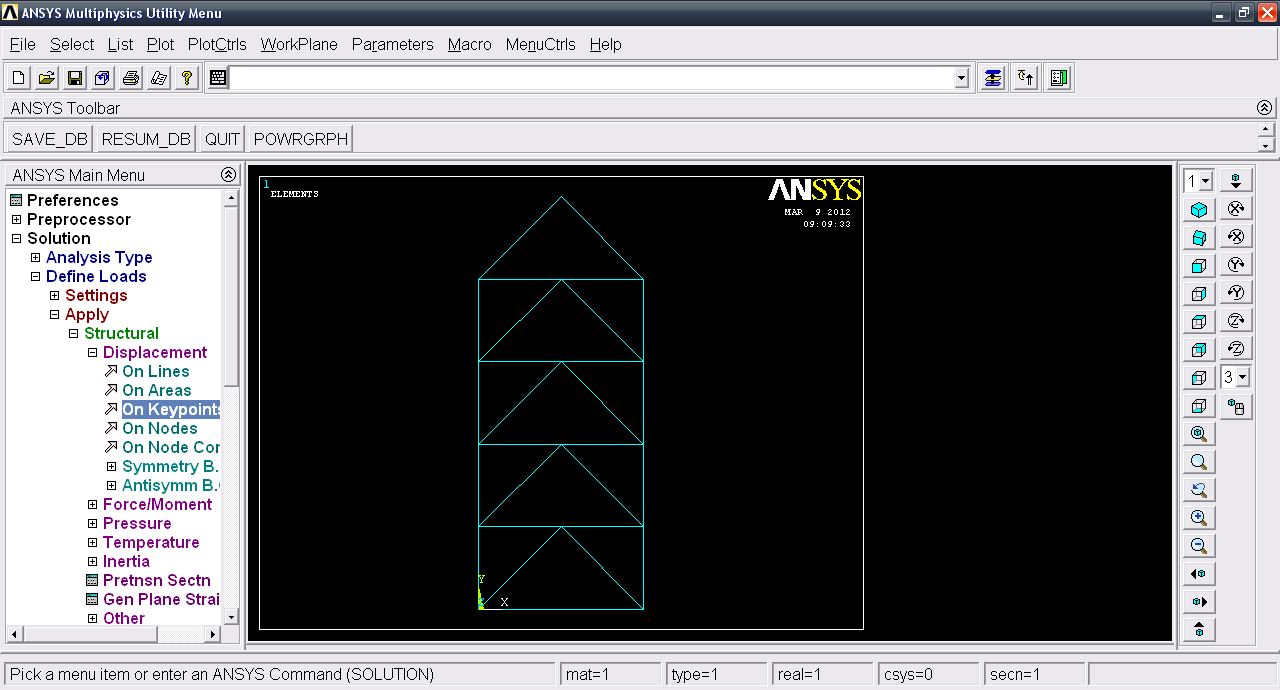22. ### Step 22:

Select two fixed lower keypoints.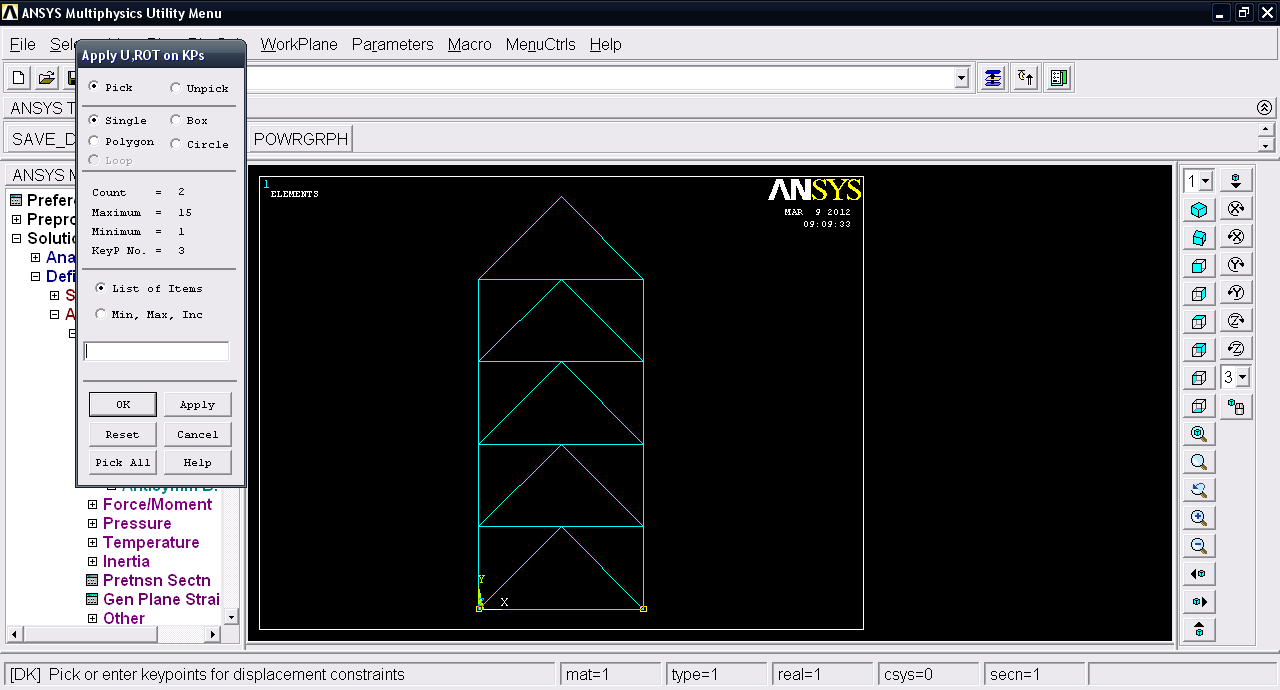23. ### Step 23:

Click OK and then select ALL DOF and click OK.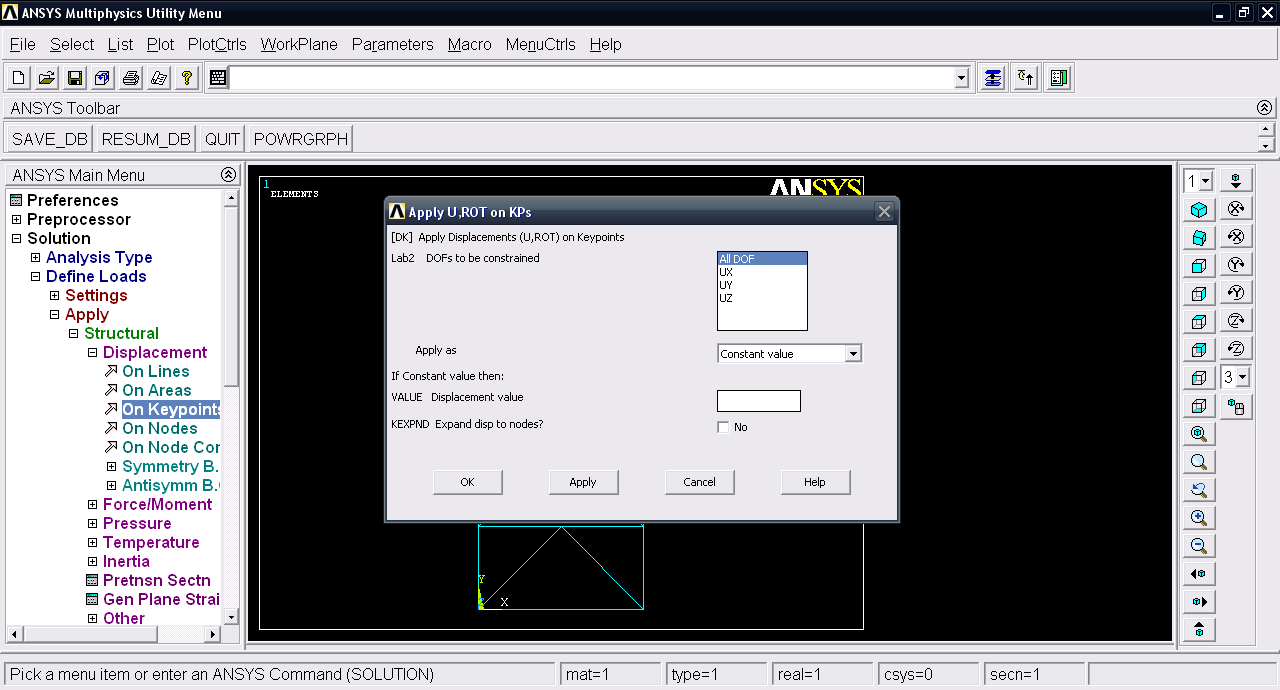24. ### Step 24:

Now Define Loads >> Apply >> Structural >> Force/Moment >> On keypoints.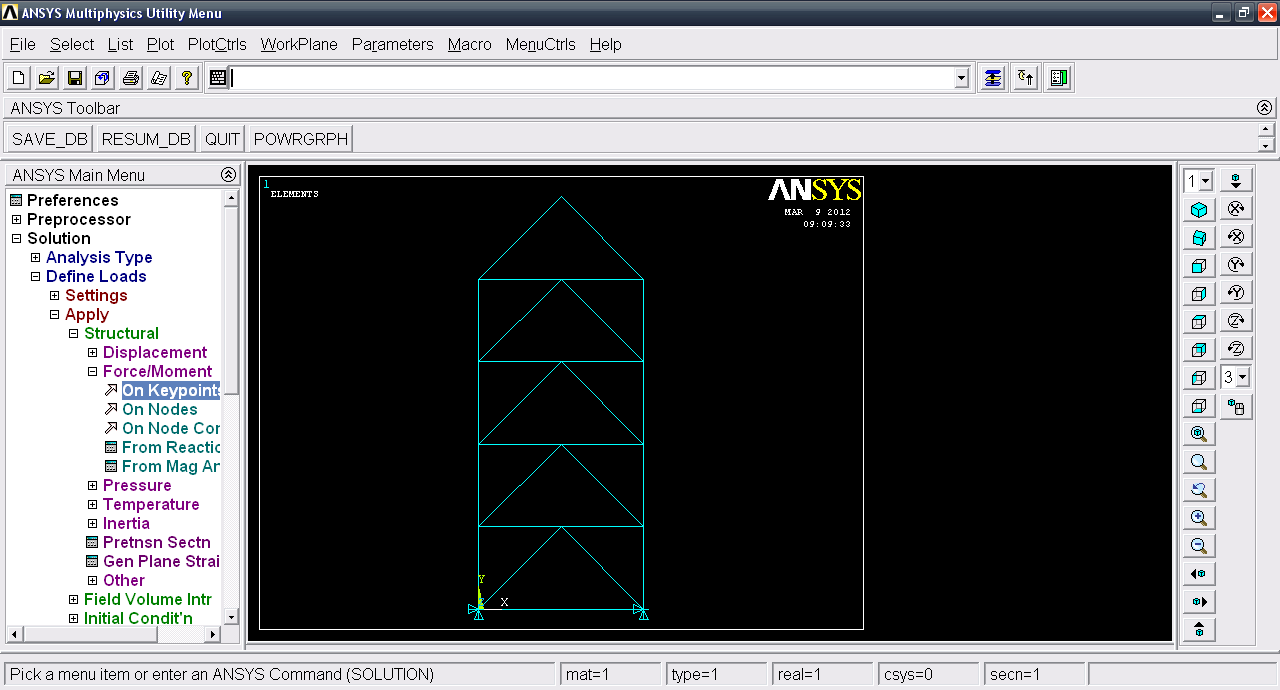25. ### Step 25:

Select top keypoints. Click OK.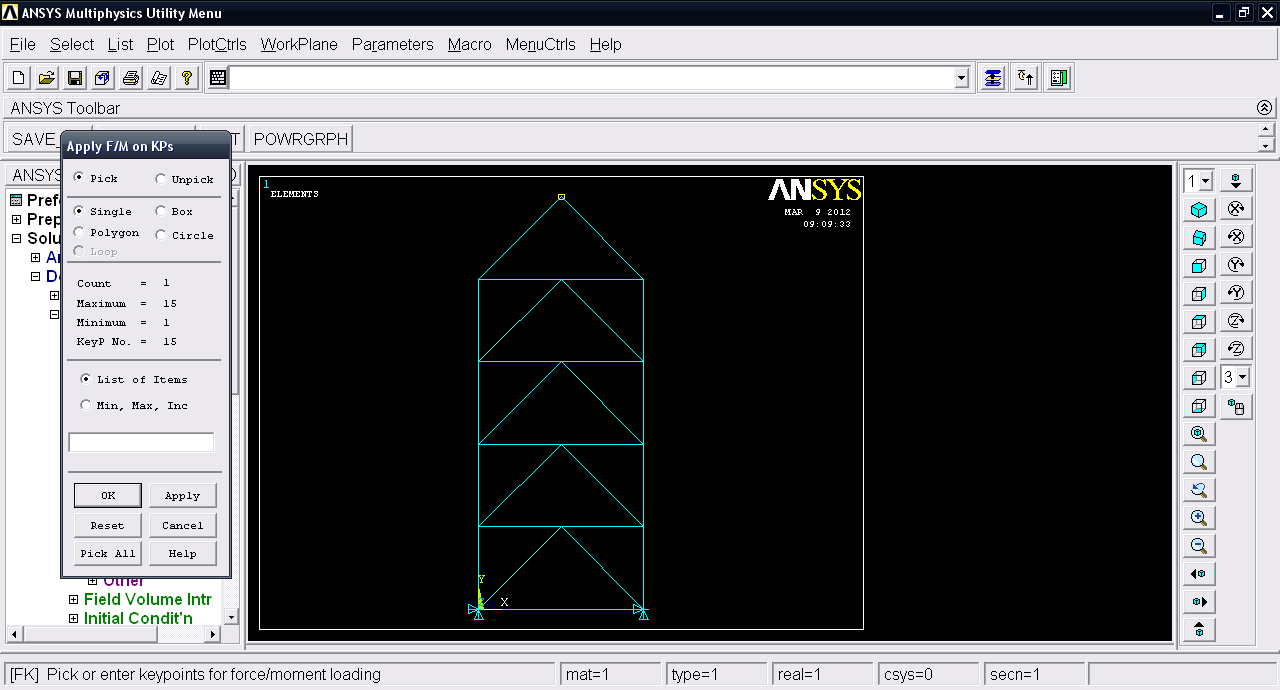26. ### Step 26:

Input force value = 5000N. Click OK.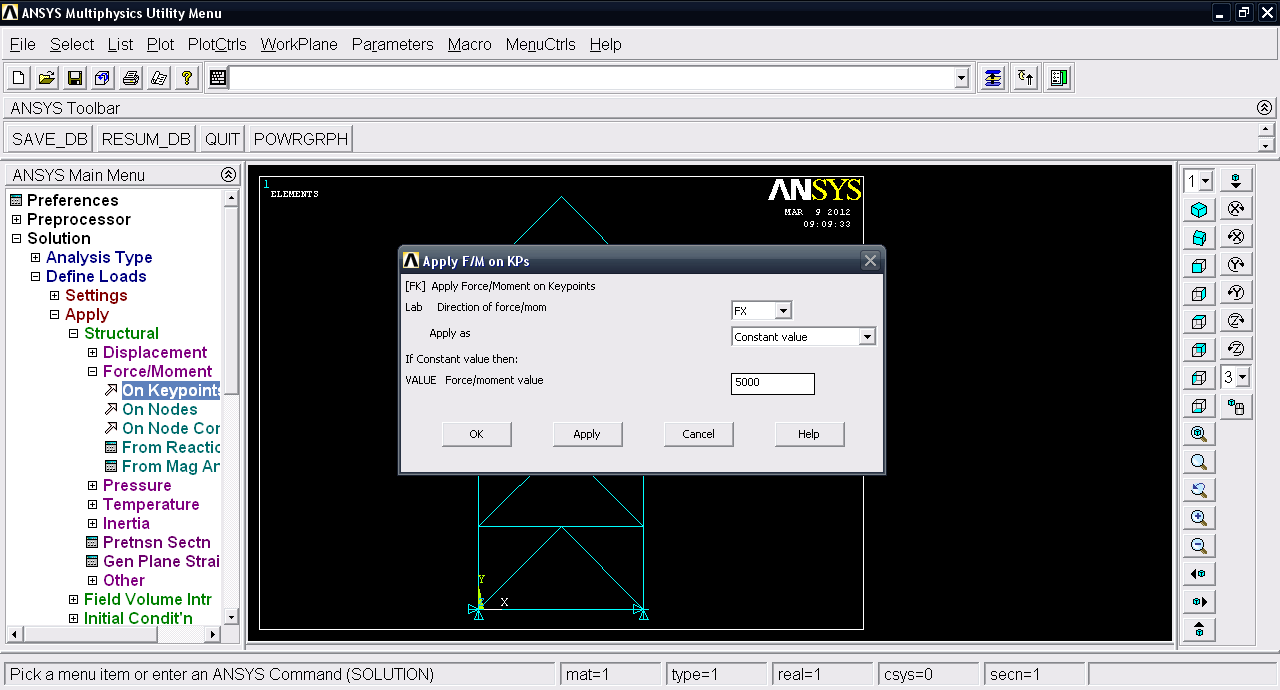27. ### Step 27:

Now save the project.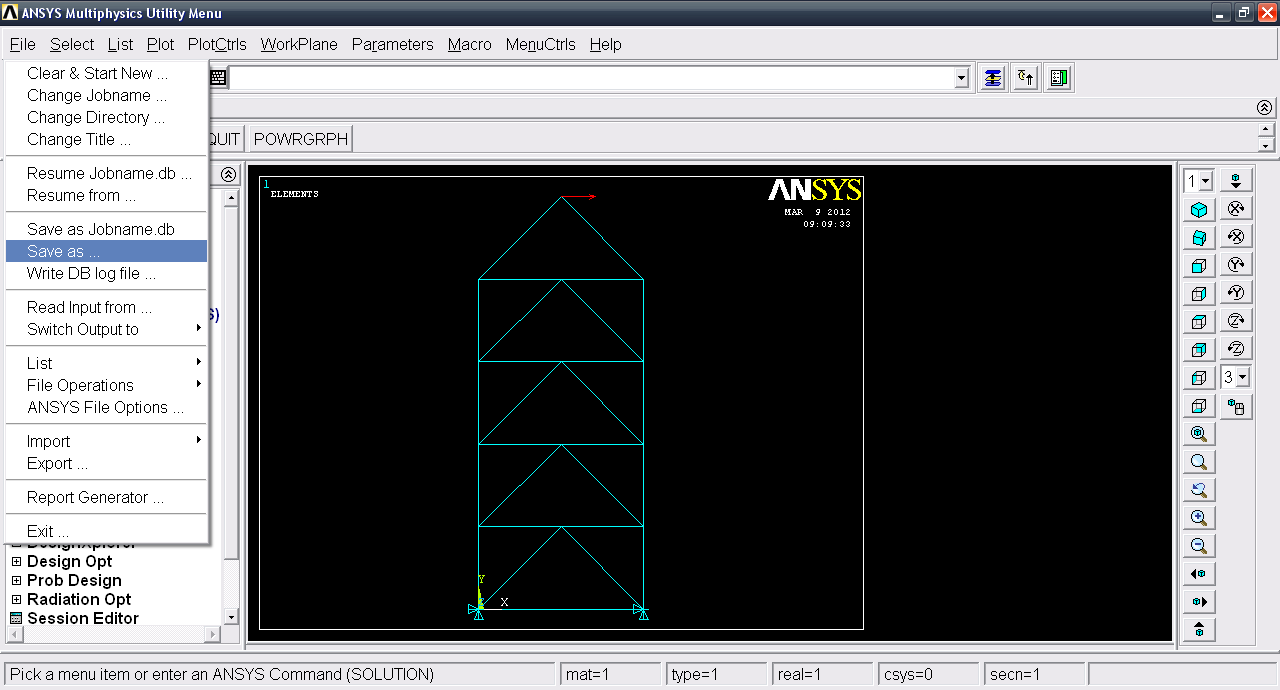28. ### Step 28:

Now second step i.e. Solution. Solution >> Solve >> Current LS.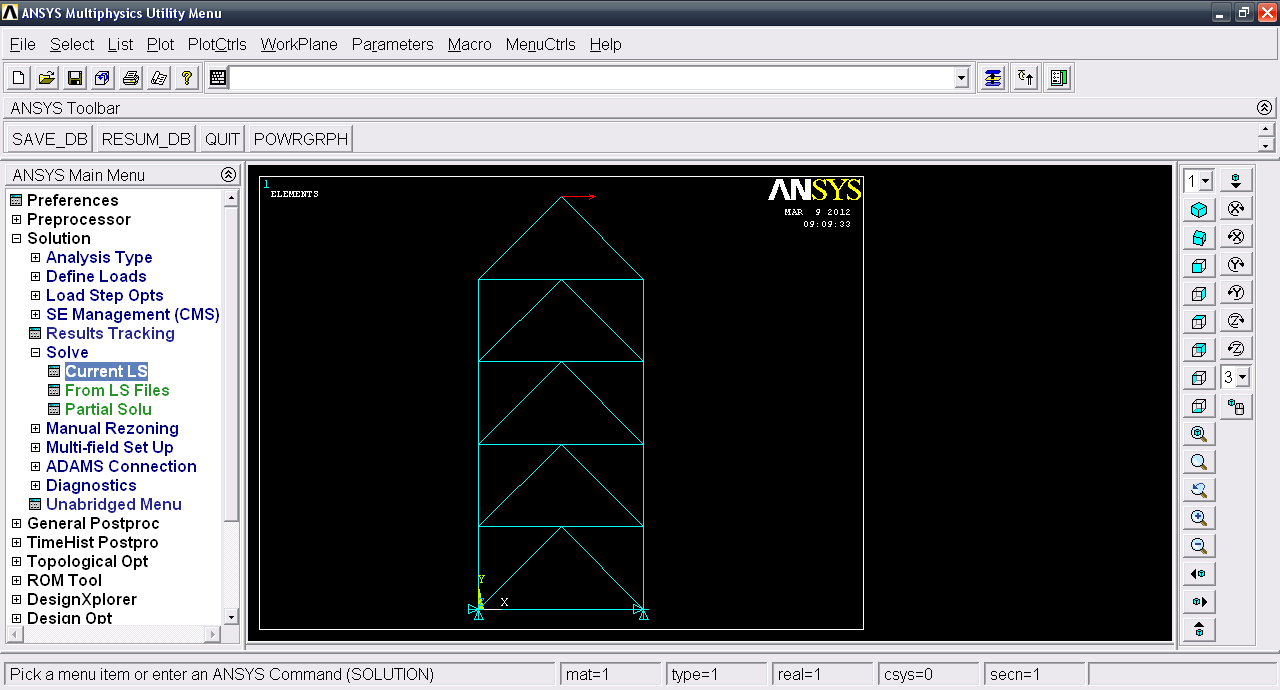29. ### Step 29:

Click OK.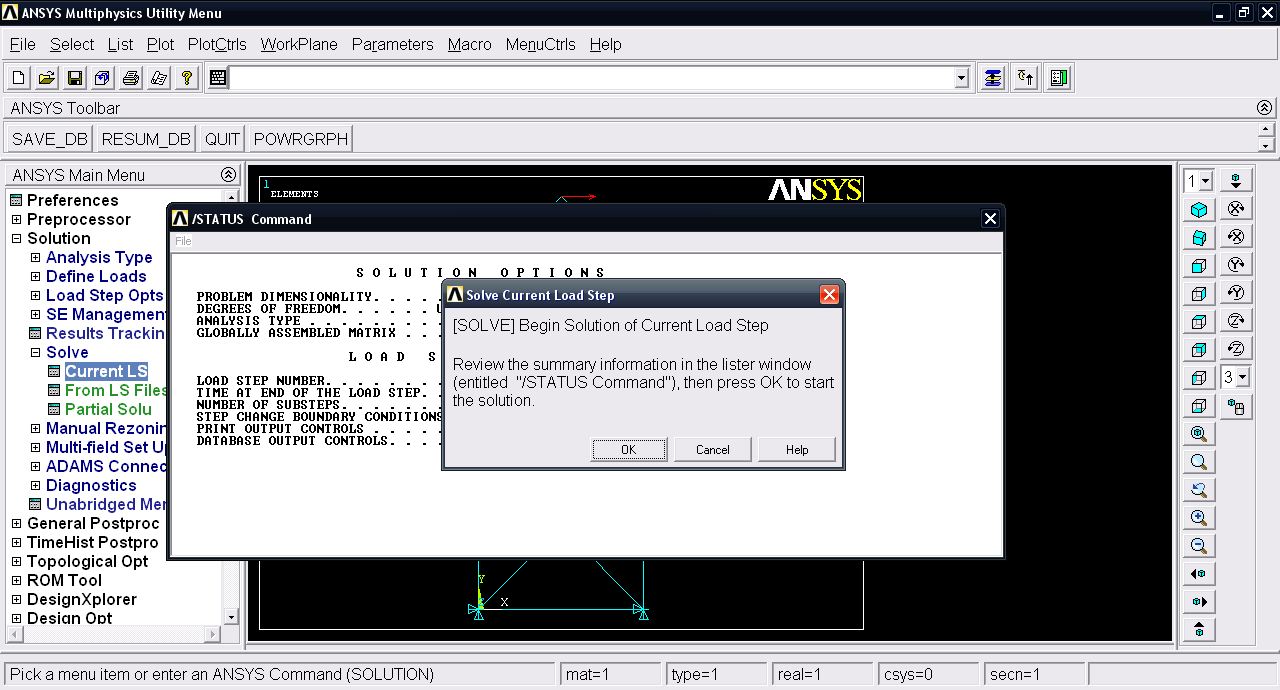30. ### Step 30:

Click Close.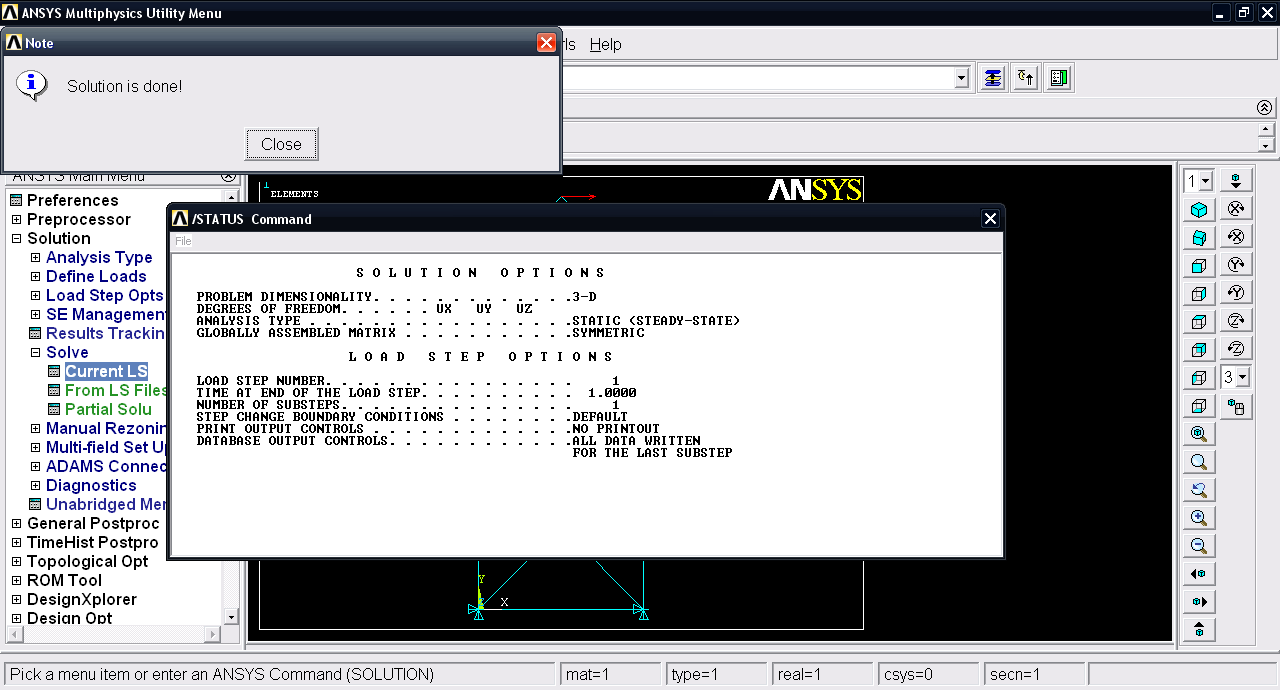31. ### Step 31:

Now third step i.e. Post proecessing. General postproc >> List results >> Reaction Solu.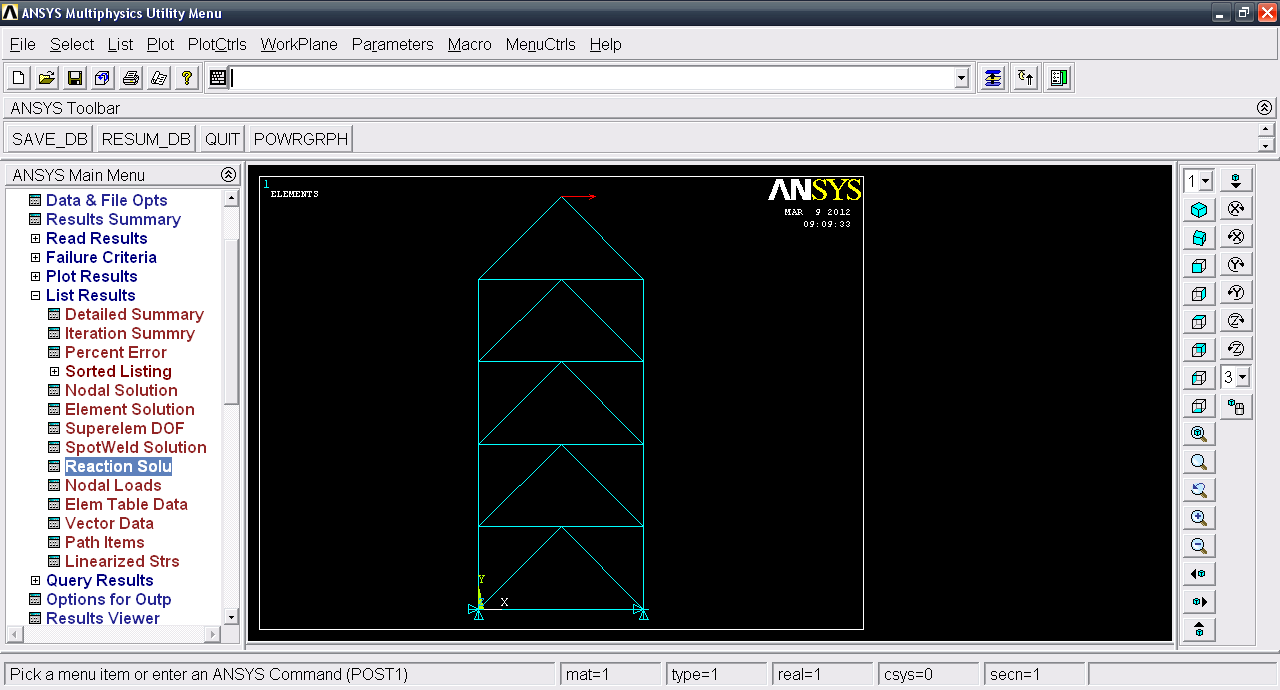32. ### Step 32:

Click OK.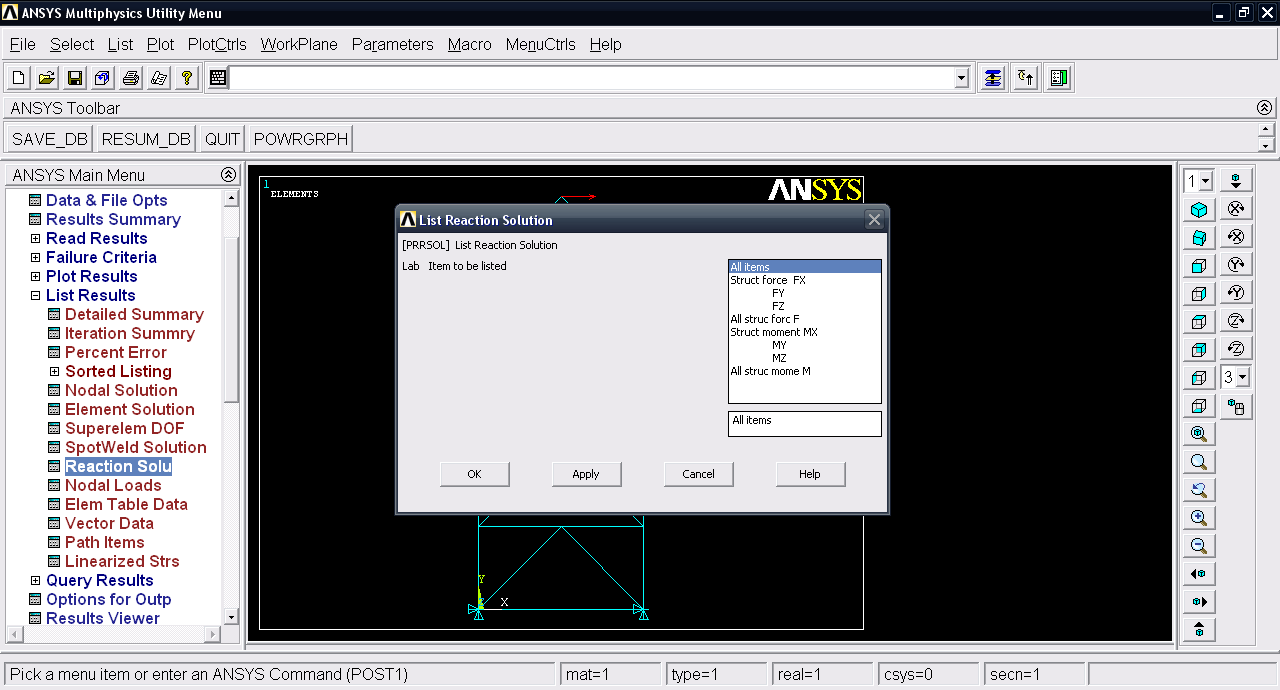33. ### Step 33:

Now we have the reaction on the 1st and 2nd node which are fixed.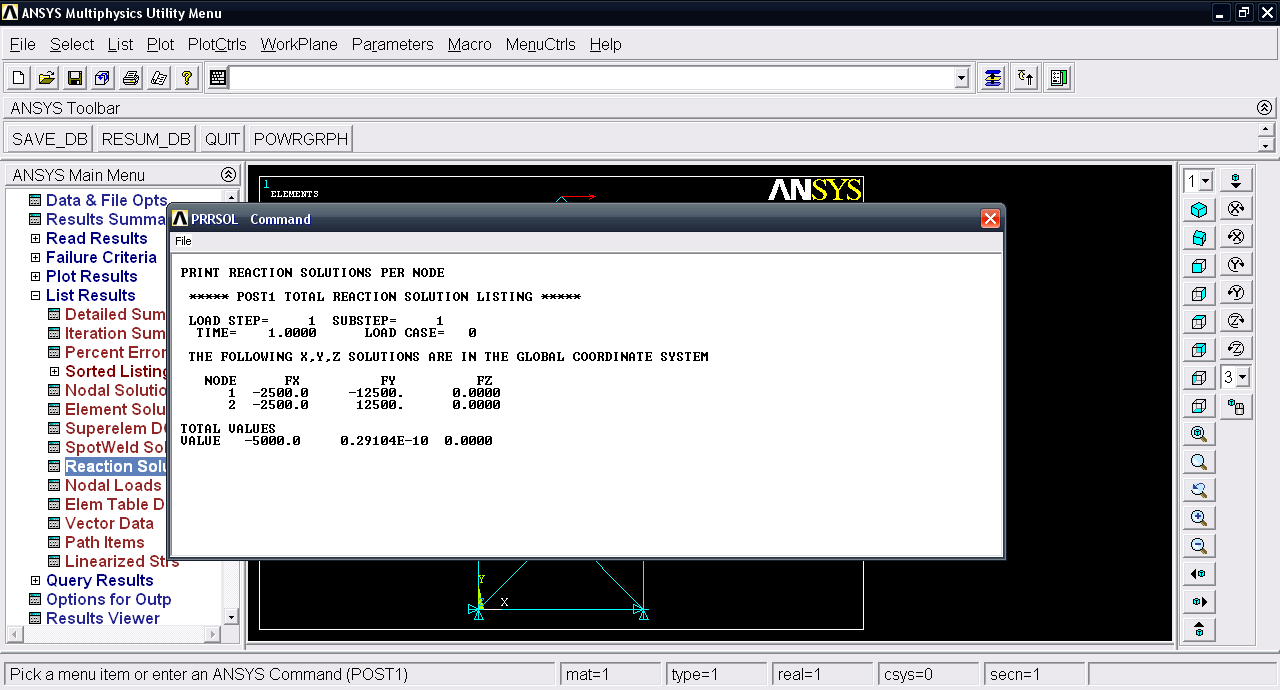34. ### Step 34:

Under Plot Results >> Deformed shape.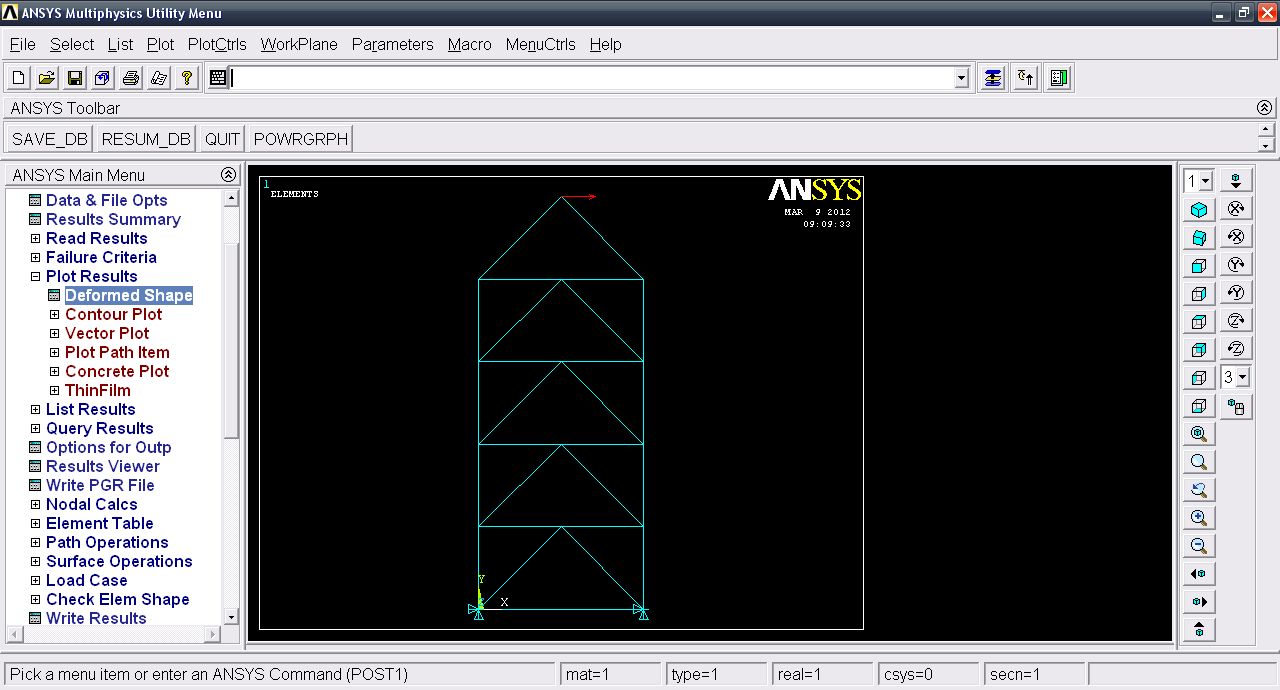35. ### Step 35:

Select Def+Undeformed and click OK.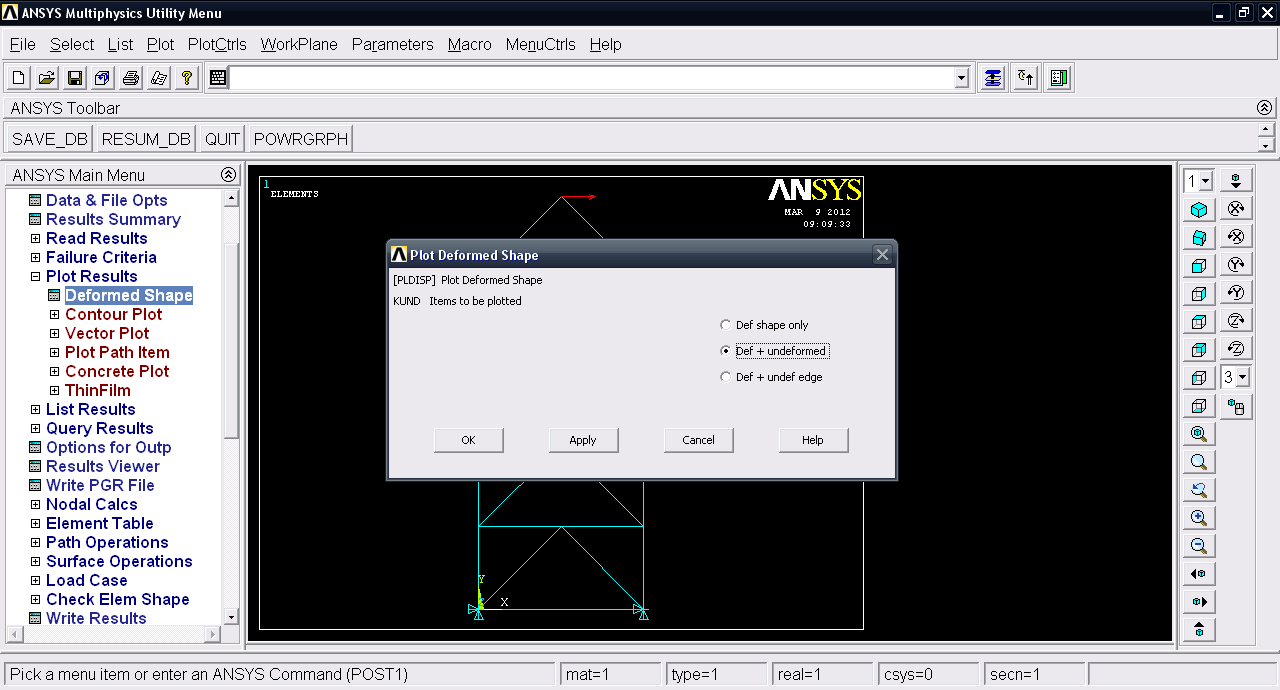36. ### Step 36:

We have deformation plot.